# Art school

Every fifth pupil 9A goes to art school. How many percent of pupils in class 9A go to art school?

Correct result:

p =  20 %

#### Solution:We would be pleased if you find an error in the word problem, spelling mistakes, or inaccuracies and send it to us. Thank you!Tips to related online calculators

## Next similar math problems:

• PupilsThere are 32 pupils in the classroom, and girls are two-thirds more than boys. a) How many percents are more girls than boys? Round the result to a whole percentage. b) How many are boys in the class? c) Find the ratio of boys and girls in the class. Writ
• StudentsAfter the fifth-grade class left 20% of students. In the seventh grade were added 2 pupils, in the eighth 1 pupil, in the ninth, the number has not changed, but it is now tenth students less than it was in the fifth grade. How many pupils are in the 9th g
• In the classroomThere are 30 boys and a few girls in the class. In the six months, 28 boys and all girls benefited, which was 95% of all pupils. How many pupils are there in the classroom?
• Dining questionnaire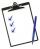In the class of 32 pupils, the class teacher delivered a satisfaction questionnaire to the school canteen. Eleven people have questioned whether they are satisfied with the dining room. The completed questionnaire also included the vote of the class teach
• Cube zoomHow many percents do we increase the volume and surface of the cube if we magnify its edge by 38 %?
• Price of state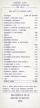Estimate the cost of the state based on following reason. State price is expressed as a percentage respectively share in the redistribution of national GDP. Employee creates monthly value € 868 (super-gross wage).Then tax him 35.2% employer contributions
• Cross-country competition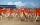Cross-country competition was attended by 76% of pupils of class 9.B. Class consis of 16 boys, two of whom did not run and 4 girls did not run. How many girls go to class 9.B?
• Geography testsOn three 150-point geography tests, you earned grades of 88%, 94%, and 90%. The final test is worth 250 points. What percent do you need on the final to earn 93% of the total points on all tests?
• IcerinkRectangular rink with dimensions of 68.7 m and 561 dm must be covered with a layer of ice 4.2 cm thick. How many liters of water is necessary for the formation of ice when the volume of ice is 9.7% greater than the volume of water.
• Bronze, tin and copper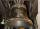Bronze is an alloy of tin and copper. An alloy of 10% tin and 90% copper is Gunmetal. If it contains 20% tin and 80% copper, it is bell metal. How many tons of molten bell metal and how many tons of copper is needed to make 100 tons of Gunmetal?
• Four classses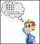Students of all 7, 8 and 9 classes in one school may take up 4,5,6 and 7 abreast and nobody will left. How many is the average count of pupils in one class if there are always four classes each grade?
• Vinegar mixing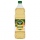We mix 70g water and 50g 8% vinegar. How many percents of the solution of vinegar we produce?
• PupilsThere are 350 girls in the school, and the other 30% of the total number of pupils are boys. How many pupils does the school have?
• Prices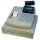The price of the product was increased by 35%. How many percents of the new price we have to make it cheaper so that its price is equal to the original price?
• Tiles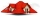The pupils of the building school have to calculate how many roof tiles they will need to cover the roof of the house in the shape of two rectangles measuring 10 x 7.2 m. One roof tile covers a rectangular area of 22 cmx32 cm. How many tiles will they nee
• ClassroomThere are eighty more girls in the class than boys. Boys are 40 percent and girls are 60 percent. How many are boys and how many girls?
• The bar chartThe bar chart shows the results of the math test. How many% of pupils achieved approximately better results than the average of the class? Diagram: 1-10 pupils, 2-4 pupils, 3-6 pupils, 4-2 pupils, 5-4 pupils. (lowest result are better)